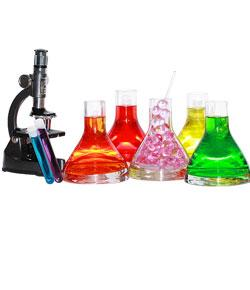# Chemistry - Semester 1 - Practice Final

14 Questions | Attempts: 7810SettingsChemistry Final. Mrs. O'Gorman. Semester 1. 2010.

• 1.
The sate of matter for an object that has definite shape and definite volume is
• A.

Solid

• B.

Liquid

• C.

Gaseous

• D.

Mixed

• E.

Elemental

• 2.
Which of the following involved a chemical change?
• A.

Digesting food

• B.

Melting ice

• C.

Boiling water

• D.

Chopping wood

• E.

None of the above

• 3.
A sample of an element contains only one kind of
• A.

Pure substance

• B.

Mixture

• C.

Isotope

• D.

Atom

• E.

None of the above

• 4.
A pure substance made up of more than one kind of atom is called
• A.

An element

• B.

A compound

• C.

A heterogenous mixture

• D.

A homogenous mixture

• E.

An alloy

• 5.
In a chemical change
• A.

A phase change must occur

• B.

A phase change never occurs

• C.

The original material can never be regenerated

• D.

The products are different substances from the starting materials

• 6.
Which of these is a chemical property?
• A.

Helium is a gas

• B.

Water has a high specific heat

• C.

Water boils at 100ªC

• D.

Neon is very nonreactive

• E.

Potassium is a soft, shiny metal.

• 7.
The symbol, Ca+1 can be used to represent
• A.

A calcium ion

• B.

A krypton ion

• C.

Potassium

• D.

A potassium atom

• E.

A krypton atom

• 8.
In chemistry, a formula is used to represent
• A.

An element

• B.

An atom

• C.

A compound

• D.

All the above

• E.

None of the above

• 9.
How many oxygen atoms are indicated in the formula Ca3(PO4)2?
• A.

6

• B.

4

• C.

13

• D.

8

• E.

None of the above

• 10.
The total number of atoms indicated by the formula Fe2(SO4)3 is
• A.

5

• B.

7

• C.

19

• D.

10

• E.

17

• 11.
The chemical formula Fe2(SO3)3 indicates
• A.

Three atoms of iron and three atoms of Sulfur

• B.

Six atoms of each element

• C.

Six atoms of oxygen

• D.

Two atoms of iron, three atoms of oxygen, one atom of sulfur

• E.

None of the above

• 12.
How many protons, electrons, and neutrons respectively does 235U have?
• A.

92, 143, 235

• B.

92, 92, 143

• C.

92, 92, 235

• D.

74, 53, 235

• E.

74, 92, 235

• 13.
Atoms of the same element having the same atomic number but different mass numbers are called
• A.

Orbitals

• B.

Isotopes

• C.

Neutrons

• D.

Isomers

• E.

Nuclei

• 14.
An element's most stable ion forms an ionic compound with chlorine having the formula XCl2. If the ion of element X has a mass of 34 and 18 electrons, what is the identity of the element, and how many neutrons does it have?
• A.

Ar, 16 neutrons

• B.

Ca, 14 neutrons

• C.

Ar, 18 neutrons

• D.

S, 18 neutrons

• E.

K, 20 neutrons

## Related TopicsBack to top
×

Wait!
Here's an interesting quiz for you.